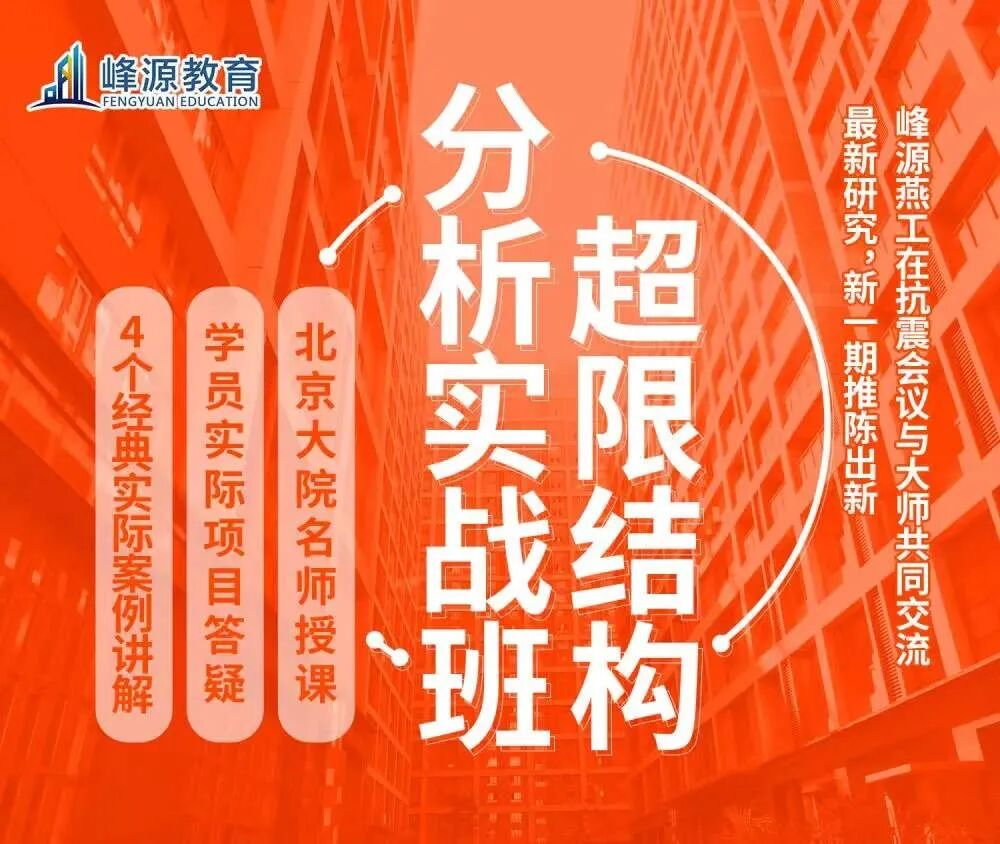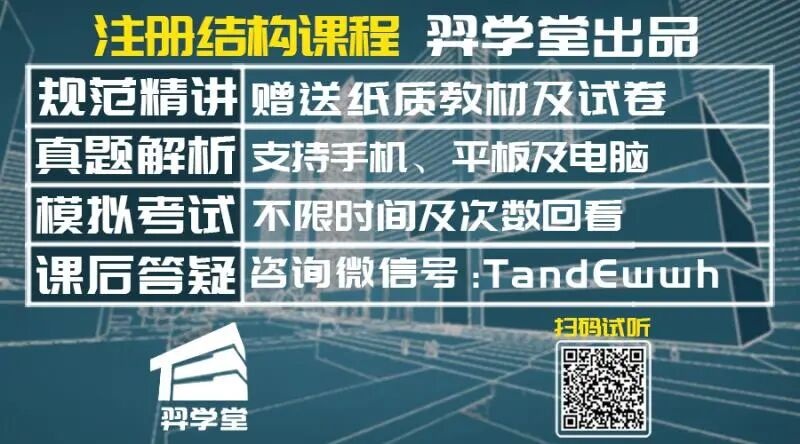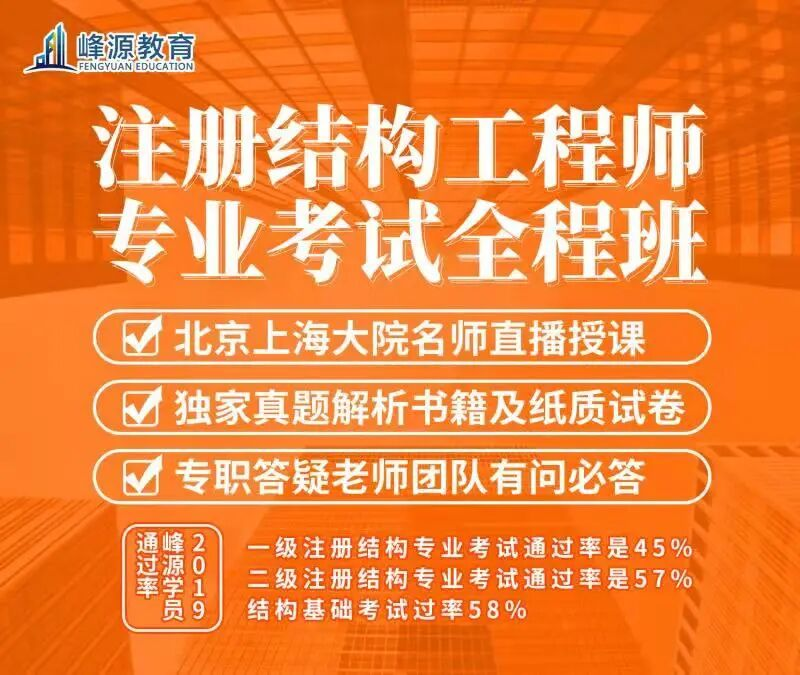# Building structure from ground motion to design spectrumChina Construction Industry Press, 2008

.As shown in Figure 3, when the frequency is small (i.e

.The spectrum value is obtained by multiplying the corresponding ground motion value by the amplification factor

.It is assumed that when the damping ratio is 2%, the intersection of the acceleration spectral line and the ground peak acceleration is at the frequency of 30 Hz, and the descent section of the spectral acceleration under other damping ratios is parallel to the descent section of the spectral acceleration with the damping ratio of 2%

.China Construction Industry Press, 2008

.2、 Response spectrum the response spectrum of a single seismic wave refers to the relationship between the maximum response (usually absolute acceleration, relative velocity and relative displacement) of a damped single degree of freedom (SDOF) system and the frequency or period of the system under a specific ground motion

.the period is long), the maximum relative displacement is large and the pseudo acceleration is small; when the frequency is large (i.e

.The design response spectrum can be obtained by smoothing a large number of response spectra of specific site conditions

.

In this theory, the building is regarded as a rigid body, and the earthquake action is simplified as the equivalent horizontal static force acting on the center of mass of the rigid body

.

In the official account of the 2019 seismic wave response spectrum attached to the source code, several numerical integration methods are explained in detail, and the source code is given

.

Figure 4: Housner with PGA normalized to 0.2g  source: fazad Naim, Naim, Wang Yayong

.

The response spectrum is normalized to zero periodic acceleration (ground acceleration) of 0.2g, and the response spectrum of other records is adjusted in proportion

.

The intersection points of spectral displacement and velocity, spectral velocity and acceleration determine the three amplification sections of the design spectrum

.

The seismic force reduction factor and displacement amplification factor are adopted in the current seismic code to consider the energy absorption capacity of the structure due to inelastic response

.

(1) 1959 code (revised in 1961) the first draft in 1959 referred to the 1957 code of the former Soviet Union, and then made significant changes in the 1961 draft, increasing the research results of Chinese scholars .

.

At the frequency of about 6Hz, the spectral acceleration decreases to the same level as the peak ground acceleration

.

They suggested that the design spectra of acceleration, velocity and displacement magnification should be kept constant in the low, medium and high frequency bands respectively (see Table 1), and the corresponding ground peak acceleration, velocity and displacement should be 1.0g, 48in / s and 36in respectively, so as to calibrate the design spectra

.

1 velocity displacement time curve obtained by recording the corrected acceleration time history and integration of eicentro wave s00e component  FIG

.

After adjusting the proportion of normalized design spectrum according to the specified acceleration, it can be used in structural design

.

Horizontal static seismic theory originated in Japan

.

Seismic design manual [M]

.

Seismic design manual [M]

.

Their proposed ground motion values and magnification are based on the analysis of several seismic records, without considering the different site conditions

.

The convolution integral of the baseline corrected seismic records can get the response spectrum of a single seismic wave

.

However, there are still some problems in dynamic time history analysis, such as high calculation cost, the calculation results depend on the selection of element and constitutive model, etc., so the response spectrum method is still the mainstream in the process of structural seismic design

.

The value of this horizontal force is equal to the weight of the building multiplied by a scale factor independent of the dynamic characteristics of the structure itself

.

If inelastic deformation is allowed in the design, given ductility coefficient, the elastic force can be reduced to obtain the design force of inelastic structure

.

With the increase of damping ratio, the shape of response spectrum will become smooth

.

Figure 5 shows the design spectrum plotted on three logarithmic coordinates

.

Source: fazad Naim, Naim, Wang Yayong

.

2 Relationship between typical acceleration, velocity and displacement magnification and frequency and period PSV and PSA and SD as well as natural vibration circle frequency ω are as follows: the response spectra of relative maximum displacement SD, pseudo velocity PSV and pseudo acceleration PSA are plotted on the same logarithmic coordinate to form triple connection Coordinate response spectrum (d-v-a spectrum)

.

In the design, the seismic inertial force is still regarded as static force, which is combined with the elastic allowable stress or limit design method, so it is convenient to apply

.

As the core concept of seismic engineering, the response spectrum theory considers the relationship between the dynamic characteristics of the structure and the ground motion excitation, and retains the original form of static theory

.

It can also be seen from the above figure that when the frequency is very low (0.05Hz or lower), the spectral displacement is close to the peak ground displacement, indicating that for the system with extremely large flexibility, the maximum displacement response is equal to the maximum ground displacement response; when the frequency is very high (25-30hz), the pseudo acceleration is close to the peak ground acceleration, indicating that for the rigid system, the absolute acceleration is equal to the ground acceleration Same as

.

3、 The following figure of design response spectrum is the average response spectrum obtained by Housner in 1959 according to eight records of four earthquakes at that time, which is the earliest design response spectrum

.

The most important function of triple reaction spectrum is to approximate the shape of design spectrum

.

As shown in the figure below: when the damping ratio is 2%, 5% and 10%, the vertical coordinate V and abscissa frequency f in the d-v-a triple reaction spectrum of EI Centro wave in Figure 3 are logarithmic scales, and the two coordinates D and a are inclined to + 45 ° and – 45 ° respectively at the abscissa

.

design spectrum in the code

.

There are several representative versions of seismic codes in China

.

The structure can be simplified as a multi degree of freedom system, and the seismic response of a structure can be decomposed into a combination of multi degree of freedom system responses according to the mode response

.

Riddell and Newmark proposed a series of coefficients as magnification reduction factors, which were multiplied by the elastic design spectrum to obtain the inelastic yield response spectrum

.

Therefore, the response spectrum can be divided into three sections: low frequency section (displacement section), medium frequency section (velocity section) and high frequency section (acceleration section)

.

The seismic design theory of structures has experienced four stages: static stage, response spectrum stage, dynamic analysis stage and behavior based seismic design theory stage

.

At present, for the general small and medium-sized buildings, the theoretical basis of the bottom shear method and the mode decomposition response spectrum method based on the response spectrum theory can meet the requirements of structural analysis

.

Figure 5: design spectrum with PGA normalized to 1.0g (Newmark and Hall)

.

IV

.

Fig

.

The shape of response spectrum of seismic records is irregular, which may contain multiple peaks and valleys

.

In response spectrum method, the seismic response of a structure can be simplified as the superposition of several mode responses

.

The response spectrum theory began in the 1930s and 1940s, and developed with the increase of strong earthquake observation records and the study of structural dynamic response characteristics

.

the period is short), the relative displacement is very small and the pseudo acceleration is relatively large; for medium frequency or period, the pseudo velocity spectrum is obviously higher than the two ends of the spectrum line

.

In the late 1960s, Newmark and hall proposed the linear form of seismic design spectrum

.

Common response spectra include displacement response spectrum (SD), pseudo velocity response spectrum (PSV) and pseudo acceleration response spectrum (PSA)

.

In 1899, in his report “investigation on overturning of brick columns and columns (artificial ground motion)”, Ohmori Fangji clearly pointed out that the seismic force P on the structure can be written in the following form: where W is the weight of the structure, k = α max / G is the seismic coefficient, that is, the maximum acceleration of the structure when it is damaged is equal to the maximum acceleration of the ground motion

.

In each section, the amplification degree of corresponding ground motion response reaches the maximum

.

The maximum response of each single degree of freedom system can be obtained from the response spectrum

.

Tags:

### Related Post## Construction qualification handling and handling process of Danjiangkou CityConstruction qualification handling and handling process of Danjiangkou City

Implement the reform of “multi drawing joint examination” of construction drawings in the whole region, develop and go online According to Ningxia construction drawing digital review system, construction drawing review## Gray space in architecture, beauty!Gray space in architecture, beauty!

Do not use commercially. 5. Guan Yongkang: the premise of realizing prefabricated construction is to “practice reverse push integrated design” ｜ forum guests.. Transferred from: none other than building (ID:## Vice President Gan Hongli gave party lectures to teachers and students of the Institute of architectureVice President Gan Hongli gave party lectures to teachers and students of the Institute of architecture

All teachers, Party members and student activists of the school of architecture attended the lecture. He deeply analyzed the spiritual connotation represented by each number and comprehensively said the rich